# Dijkstra

2022/6/13

dijkstra的本质是贪心，对于求目标两个位置间的最短权边和，查找从起点位置到每个点的最短距离，同时更新与该当前点相关的点的距离。

struct EDGE
{
int next;
int to;
}edge;


int head;


int cnt=0;//指针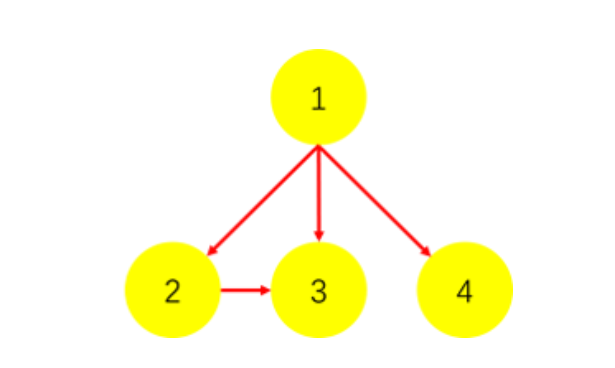1 2
1 3
1 4
2 3


1.输入1 2，代表1连向2。

cnt++;//作为结构体下标，没有意义
edge[cnt].to=2;结点1的儿子是2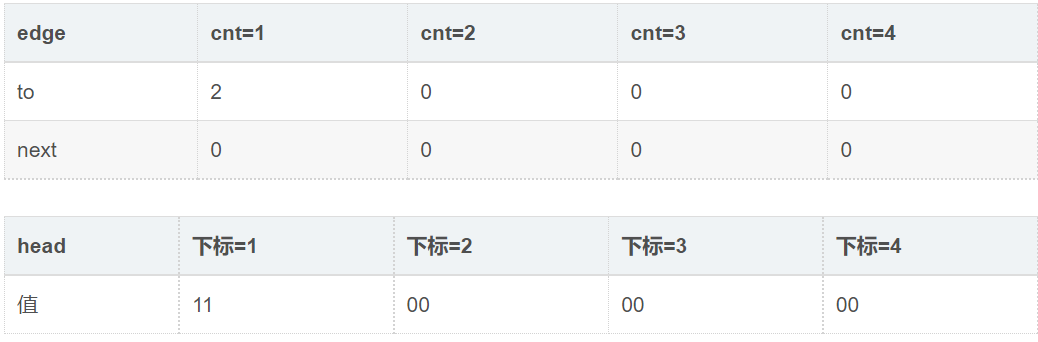2.输入1 3，代表1连向3。

cnt++;
edge[cnt].to=3;结点1的儿子是3
//这时,3成为了结点1的儿子，不过2被挤了下去...
//所以要引入结构体中next元素，记录：3还有个兄弟（next）是2
//所以代码要换成：
cnt++;
edge[cnt].to=3;//结点1连向3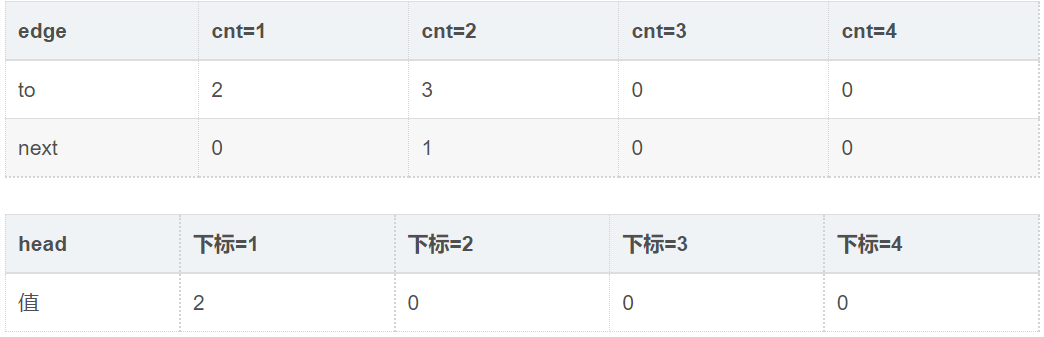3.输入1 4，代表1连向4。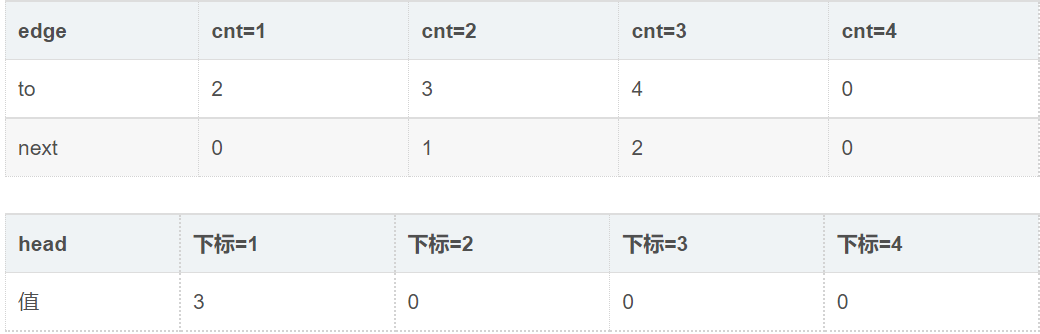4.输入2 3，代表2连向3。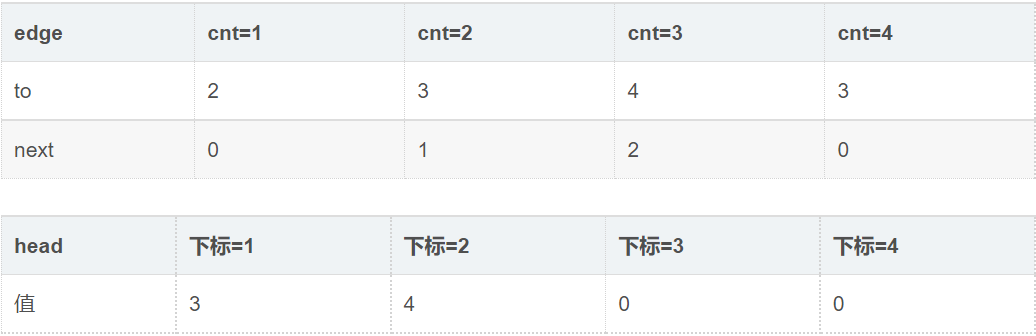#include<iostream>
using namespace std;
struct edge
{
int next;
int to;
int wei;
}edge[MAXM];
int cnt=0;
void addedge(int u,int v,int w) //起点，终点，权值
{
edge[cnt].to=v;
edge[cnt].w=w;
}
int main()
{
int n;
for(int i=1;i<=n;i++)
{
int a,b,wei;
}
}


    for(int i=head[x];i!=0;i=edge[i].next)


#include <bits/stdc++.h>
#define rep(i,n,m) for(int i=n;i<=m;++i)
#define per(i,n,m) for(int i=n;i>=m;--i)
#define INF 0x3f3f3f3f
using namespace std;
typedef long long ll;
const int N=250010;
//to记录当前边指向的结点，w记录权值，dist记录目标起点到各个点的最短距离
bool st[N];  //记录当前结点是否已经找到最短距离
int n,m,s,cnt=0;  //cnt是记录每次数据的指针，相当于下标
w[++cnt]=c;
to[cnt]=b;
}
void Dijkstra()
{
priority_queue<pair<int,int>,vector<pair<int,int>>,greater<pair<int,int>>> heap;
//优先队列自带的排序函数greater，使得默认按第一个元素升序排序
heap.push({0,s});dist[s]=0;  //s记录的是所求起点，将其放入堆中，距离为0，终点是其自己
while(!heap.empty()){
pair<int,int> temp=heap.top();
heap.pop();     //弹出权值最小的点，待处理
int x=temp.first,y=temp.second;
if(st[y])continue;  //如果该点已经找到最短距离，则跳过
st[y]=true;  //更新该点已经找到最短距离
int t=to[i];
if(dist[t]>x+w[i]){  //找到连接的可更新的最短结点
dist[t]=x+w[i];  //更新权值路径
heap.push({dist[t],t});  //把该更新过的结点放到堆中待处理
}
}
}
}
int main()
{
cin>>n>>m;
memset(dist,INF,sizeof(dist)); //初始化距离无穷大
for(int i=1;i<=m;++i){
int a,b,c;cin>>a>>b>>c;